Note: The other languages of the website are Google-translated. Back to English

## How to multiply two columns and then sum in Excel?

In Excel, most of us may suffer to multiply two columns and then add them up, of course, we can multiply each items and then sum them, but this will be troublesome if there are hundreds or thousands rows need to calculate. In Excel, there is a powerful function – SUMPRODUCT, with it, we can quickly multiply two columns and then sum them. This article, I will talk about how to apply this function.

Multiply two columns and then sum without criteria

Multiply two columns and then sum based on one or more criteria

Multiply two columns and then sum based on one condition with a useful feature

#### Multiply two columns and then sum without criteria

Supposing you have the following data range which you need to multiply the quantity column and unit price and then add up all the multiply results together to get the total price: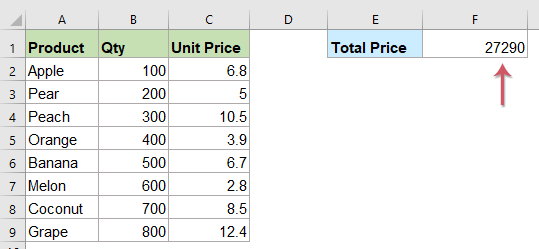Here, you can apply the SUMPRODUCT function to solve it, please enter the following formula into a cell where you want to output the result:

=SUMPRODUCT(B2:B9,C2:C9)

Note: In the above formula, B2:B9, C2:C9 are the two columns range that you want to do the calculation.

And then press Enter key to get the result, see screenshot: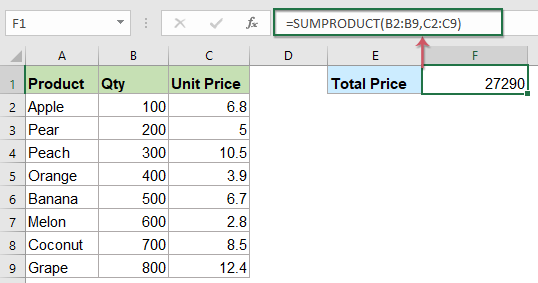#### Multiply two columns and then sum based on one or more criteria

##### Multiply two columns and then sum with one criterion

In the following worksheet data, I just want to get the total price of apple, so we can expand the SUMPRODUCT function, please enter this formula:

=SUMPRODUCT(\$B\$4:\$B\$12,\$C\$4:\$C\$12*(\$A\$4:\$A\$12=E4))

Note: In the above formula, B4:B12, C4:C12 are the two columns range that you want to do the calculation, E4 is the criteria that you want to calculate based on, and A4:A12 is the column data contains the condion you look for.

Then press Enter key to return the calculation result: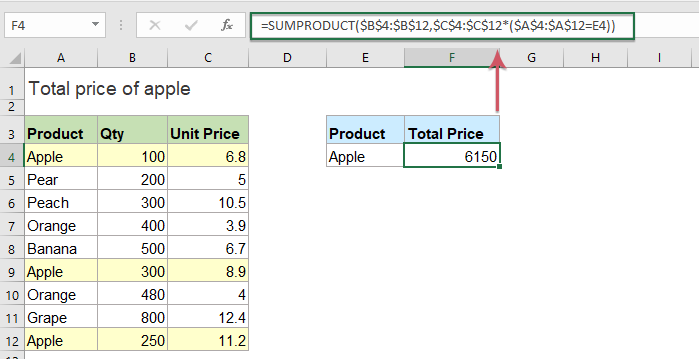##### Multiply two columns and then sum with more criteria

1. Multiply two columns and then sum based on multiple criteria in multiple columns

We can also use the SUMPRODUCT function to multiply two columns and add up the values based on more criteria, for instance, I want to calculate the total price of apples are supplied by China. Please copy or enter the below formula into a blank cell:

=SUMPRODUCT(\$C\$4:\$C\$12*(\$A\$4:\$A\$12=F4),\$D\$4:\$D\$12*(\$B\$4:\$B\$12=G4))

Note: In the above formula, C4:C12, D4:D12 are the two columns range that you want to do the calculation, F4, G4 are the criteria that you want to calculate based on, and A4:A12, B4:B12 are the columns contain the conditions you specified.

And then, press Enter key to get the result as below screenshot shown: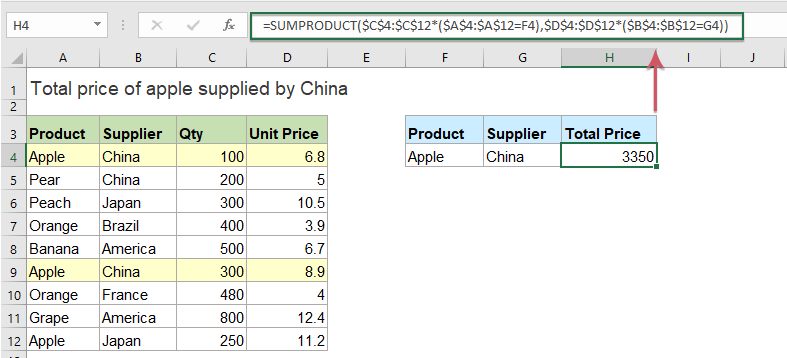2. Multiply two columns and then sum based on multiple criteria in the same column

If I need to calculate the total price of apples and oranges, please use this formula:

=SUMPRODUCT(((\$A\$4:\$A\$12=F4)+(\$A\$4:\$A\$12=F5)),\$C\$4:\$C\$12,\$D\$4:\$D\$12)

Note: In the above formula, C4:C12, D4:D12 are the two columns range that you want to do the calculation, F4, F5 are the criteria that you want to calculate based on, and A4:A12, isthe columns contains the conditions you specified.

And then, press Enter key to get the result as below screenshot shown: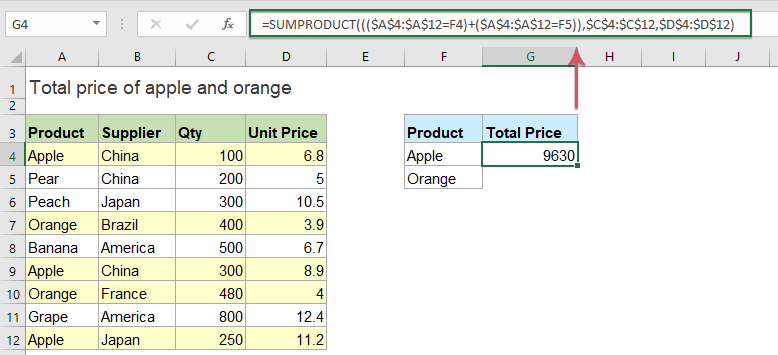Tips: The above examples are some basic formulas, you can add more criteria as you need within the basic formula.

#### Multiply two columns and then sum based on one condition with a useful feature

To multiply two columns and then sum the result based on one conditon,Kutools for Excel's SUMPRODUCT with criteria feature also can do you a favor.

Note:To apply this Date & Time Helper, firstly, you should download the Kutools for Excel, and then apply the feature quickly and easily.

After installing Kutools for Excel, please do as this:

1. Click a cell where you want to put the result, and then click Kutools > Formula Helper > Formula Helper, see screenshot: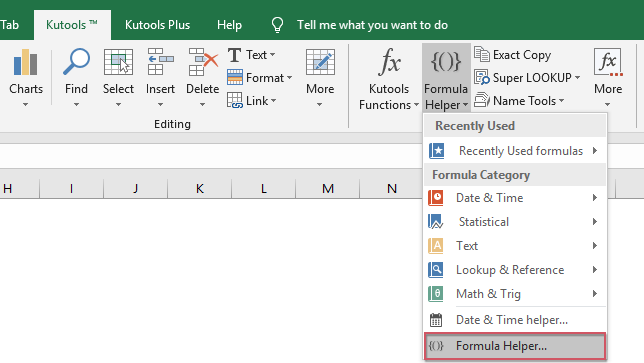2. In the Formulas Helper dialog box, do the following operations:

• Select Math from the Formula Type drop down list;
• In the Choose a formula listbox, select SUMPRODUCT with criteria option;
• Then, in the Arguments input section, select the Lookup_col, Lookup_value, Array 1 and Array 2 from the original table as you need.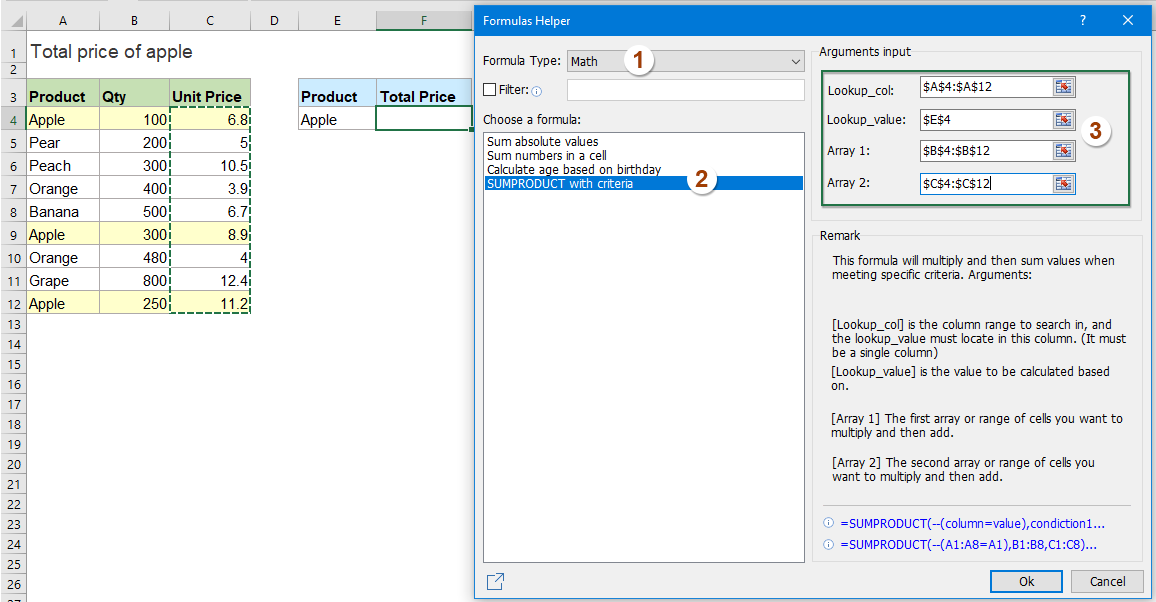3. Then, click Ok button, and the calculated result will be displayed at once, see screenshot: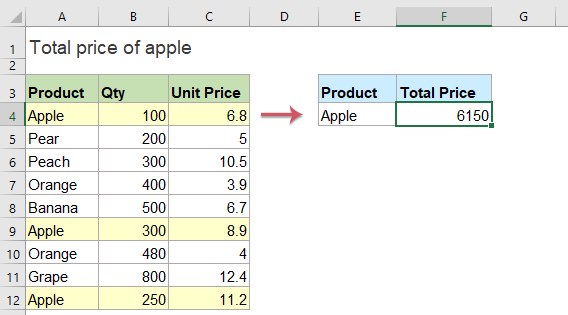Kutools for Excel's Formula Helper contains dozens of common used formulas such as Date & Time, Lookup & Reference and so on helps you to create some useful formulas without remembering any formulas which can save much time in your daily work. Click to download Kutools for Excel!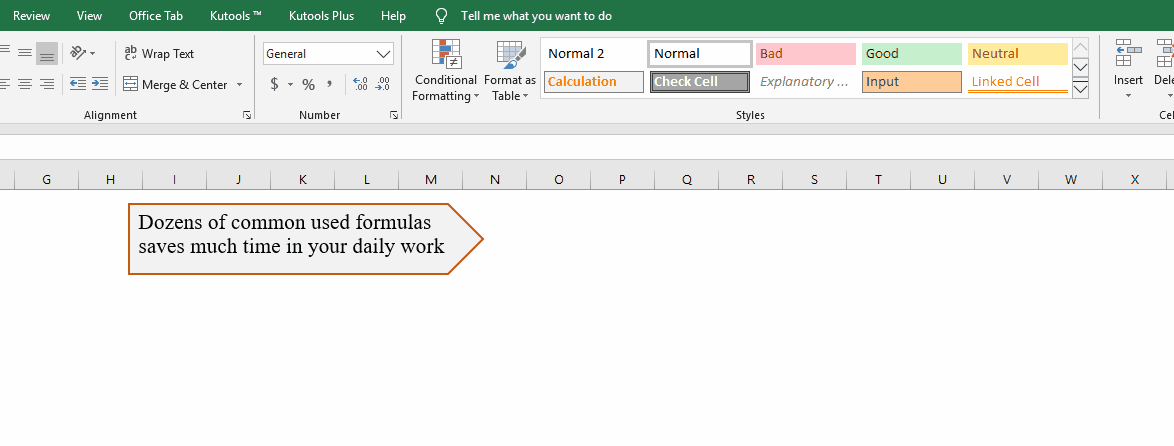Kutools for Excel: with more than 300 handy Excel add-ins, free to try with no limitation in 30 days. Download and free trial Now!

### The Best Office Productivity Tools

#### Kutools for Excel Solves Most of Your Problems, and Increases Your Productivity by 80%

• Reuse: Quickly insert complex formulas, charts and anything that you have used before; Encrypt Cells with password; Create Mailing List and send emails...
• Super Formula Bar (easily edit multiple lines of text and formula); Reading Layout (easily read and edit large numbers of cells); Paste to Filtered Range...
• Merge Cells/Rows/Columns without losing Data; Split Cells Content; Combine Duplicate Rows/Columns... Prevent Duplicate Cells; Compare Ranges...
• Select Duplicate or Unique Rows; Select Blank Rows (all cells are empty); Super Find and Fuzzy Find in Many Workbooks; Random Select...
• Exact Copy Multiple Cells without changing formula reference; Auto Create References to Multiple Sheets; Insert Bullets, Check Boxes and more...
• Extract Text, Add Text, Remove by Position, Remove Space; Create and Print Paging Subtotals; Convert Between Cells Content and Comments...
• Super Filter (save and apply filter schemes to other sheets); Advanced Sort by month/week/day, frequency and more; Special Filter by bold, italic...
• Combine Workbooks and WorkSheets; Merge Tables based on key columns; Split Data into Multiple Sheets; Batch Convert xls, xlsx and PDF...
• More than 300 powerful features. Supports Office/Excel 2007-2019 and 365. Supports all languages. Easy deploying in your enterprise or organization. Full features 30-day free trial. 60-day money back guarantee.#### Office Tab Brings Tabbed interface to Office, and Make Your Work Much Easier

• Enable tabbed editing and reading in Word, Excel, PowerPoint, Publisher, Access, Visio and Project.
• Open and create multiple documents in new tabs of the same window, rather than in new windows.
• Increases your productivity by 50%, and reduces hundreds of mouse clicks for you every day!